# 2020 图像超分最新综述及上采样技术一览

## 目录

• Literature Review for Super Resolution

• 渐进式方法1：Laplacian金字塔超分辨率网络

• 渐进式方法2：ProSR：完全渐进式超分辨率网络

## 1 Literature Review for Super Resolution

### 1.1 超分问题的定义

$$I_x=D(I_y; \delta)\tag{1}$$

$$D(I_y; \delta)=(I_y)\downarrow_s,\left{ s \right}\subset\delta\tag{2}$$

$$D(I_y; \delta)=(I_y\otimes \kappa)\downarrows+n\varsigma,\left{ \kappa,\varsigma,s \right}\subset\delta\tag{3}$$

$$\hat{I_y}=F(I_x;\theta)\tag{4}$$

$$\hat \theta= \mathop{\arg\min}_{\theta}L(\hat{I_y}, I_y)+\lambda\Phi(\theta)\tag{5}$$

### 1.2 超分问题的数据集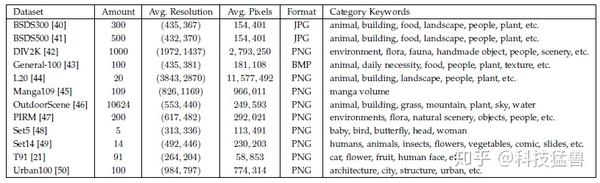### 1.3 超分问题的质量评估方法

• full-reference methods：利用相关的图片评价
• reduced-reference methods：利用提取的特征评估
• no-reference methods：blind IQA，不使用相关的图片

1. 峰值信噪比 Peak signal-to-noise ratio (PSNR)：

$$PSNR=10\cdot \log{10}(\frac{L^2}{\frac{1}{N}\sum{i=1}^{N}{I(i)-\hat{I}(i)^2}})\tag{6}$$

2. 结构相似度 SSIM

$\muI=\frac{1}{N}\sum{i=1}^{N}{I(i)}\tag{7}$ $\sigmaI=(\frac{1}{N-1}\sum{i=1}^{N}{(I(i)-\mu_I)^2})^{\frac{1}{2}}\tag{8}$

$$C_l(I,\hat I)=\frac{2\muI\mu{\hat I}+C_1}{\muI^2+\mu{\hat I}^2+C_1}\tag{9}$$

$$C_c(I,\hat I)=\frac{2\sigmaI\sigma{\hat I}+C_1}{\sigmaI^2+\sigma{\hat I}^2+C_1}\tag{10}$$

$$\sigma{I,\hat I}=\frac{1}{N-1}\sum{i=1}^{N}{(I(i)-\muI)(\hat I(i)-\mu\hat I)}\tag{11}$$

$$Cs(I,\hat I)=\frac{\sigma{I,\hat I}+C_3}{\sigmaI\sigma{\hat I}+C_3}\tag{12}$$

SSIM公式可以表示为亮度、对比度和结构比较的加权乘积，分别计算。

$$SSIM(I,\hat I)=[C_l(I,\hat I)]^\alpha[C_c(I,\hat I)]^\beta[C_s(I,\hat I)]^\gamma\tag{13}$$

3 Mean Opinion Score

### 1.4 超分网络的4种框架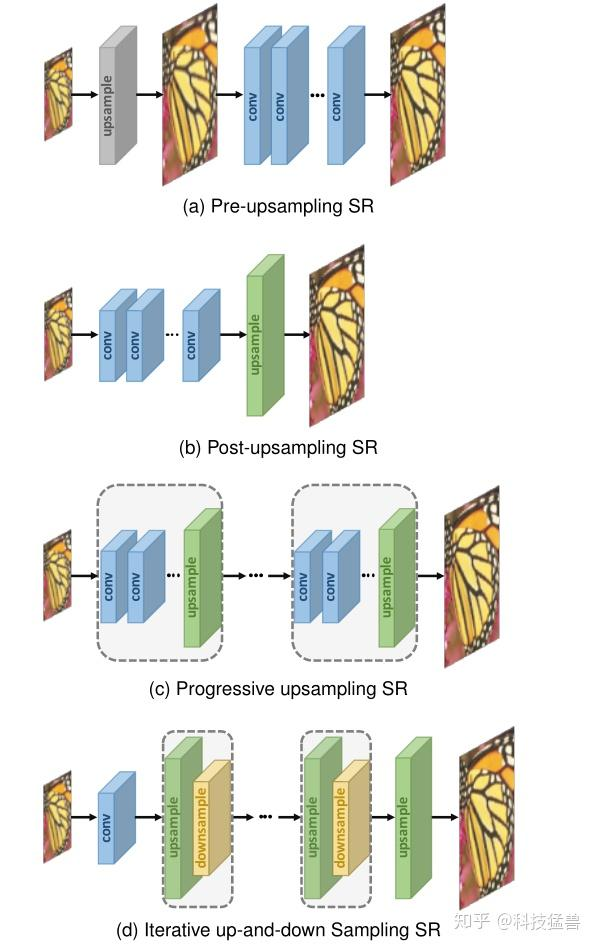• 1 Pre-upsampling Super-resolution：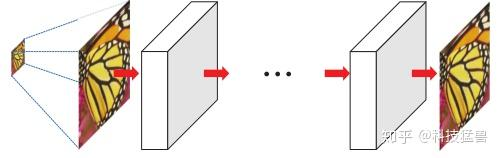ICCV 2015： Deep networks for image super-resolution with sparse prior
VDSR ICCV 2016： Accurate image super-resolution using very deep convolutional networks
DRCN CVPR 2016： Deeply-recursive convolutional network for image super-resolution
DRRN ICCV 2017： Image super-resolution via deep recursive residual network

• 因为最困难的上采样操作交给了传统方法来解决，深度神经网络只需要来对粗糙的生成图片进行精调，所以说大大降低了训练的难度。
• 这种做法可以得到任意大小的HR图片，处理任意维度的LR图片，并灵活调节超分任务的scale factor。

• 传统的上采样操作会带来很多副作用，比如噪声放大和模糊的问题。
• 增加了不必要的计算成本，并且不提供用于重建高分辨率图像的附加高频信息。

• 2 Post-upsampling Super-resolution：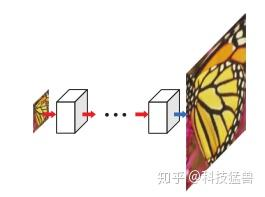CVPR 2016： Real-time single image and video super-resolution using an efficient sub-pixel convolutional neural network
CVPR 2017： Enhanced deep residual networks for single image super-resolution
ECCV 2016： Accelerating the super-resolution convolutional neural network

• 上采样仅在一个步骤中执行，那么当放大倍数比较大时(4，8)，极大地增加了学习难度。
• 每一种放大因子都需要训练一个独立的模型，无法满足多尺度超分任务的需求。

• 3 Progressive upsampling Super-resolution：

CVPR 2017： Deep Laplacian Pyramid Networks for Fast and Accurate Super-Resolution
TPAMI 2018： Fast and accurate image super-resolution with deep laplacian pyramid networks(上篇的扩大版)
CVPR 2018： A Fully Progressive Approach to Single-Image Super-Resolution

• 通过把复杂的上采样任务分解为几步进行，渐进式操作减少了学习的困难，尤其是在上采样比较多的情况。

• 多阶段上采样导致模型的设计复杂，存在很多种不同的情况，以及训练稳定性的问题。
• 需要更加复杂，先进的训练策略和指导(详见后文介绍的2篇工作)。

• 4 Iterative Up-and-down Sampling Super-resolution：

DBPN CVPR 2018： Deep back-projection networks for super-resolution
SRFBN CVPR 2019： Feedback network for image super-resolution
RBPN CVPR 2019： Recurrent back-projection network for video super-resolution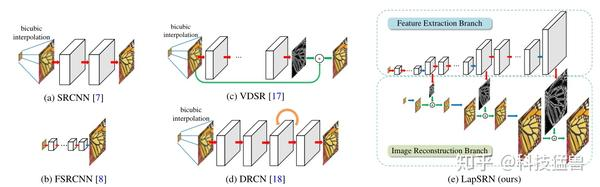### 1.5 常见的上采样方法

1.5.1 插值法：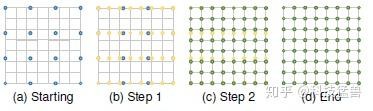1.5.2 可学习的上采样方法：

• 转置卷积层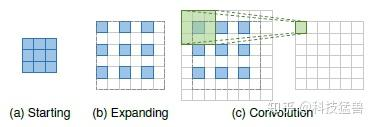• 亚像素层sub-pixel layer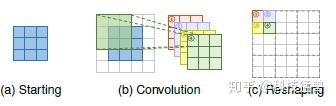• Meta upscale module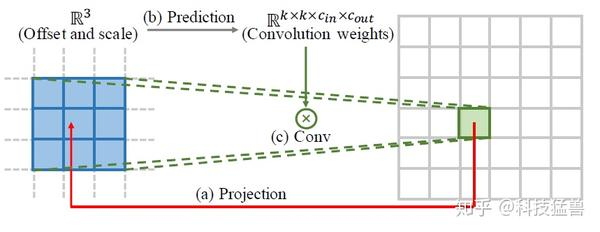$$I^{SR}(i,j)=\Phi(F^{LR}(i_l,j_l), W(i,j))\tag{14}$$

Location Projection 就是对于HR图像中的每个像素点 $(i,j)$ , 均需找到 $F^{LR}$ 中的一个像素点$(i_l,j_l)$与之相对应，即： $(i_l,j_l)=T(i,j)=(\lfloor \frac{i}{r} \rfloor, \lfloor \frac{j}{r} \rfloor)$。

Weights Prediction是利用一个网络预测上采样网络的权重。 对于传统的可学习的上采样方法，不同的尺度因子都有与之对应的、从训练集中学到的卷积核$W$。但是，本文的Meta-Upscale Module利用神经网络来预测weights。

Feature Mapping：在得到$F^{LR}$ 、利用weight prediction network得到卷积核后，要做的最后一件事情就是将权重作用在feature map上面，即：mapping feature to the value of the pixel on the HR image。公式如下：

$$\Phi(F^{LR}(i_l,j_l),W(i,j))=F^{LR}(i_l,j_l)\cdot W(i,j)\tag{15}$$

### 1.6 常见的网络架构设计

1.6.1 Residual Learning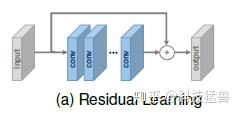1.6.2 Recursive Learning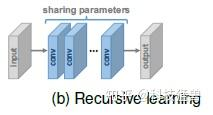DRCN采用16次单卷积层递归，感受野达到41×41，远大于SRCNN的13×13，并且没有过多参数。

DRRN将残差块作为递归单元进行25次递归，性能优于17个残差块的baseline。

MemNet是基于记忆块而设计，记忆块由6个recursive ResBlock组成，每个递归的输出拼起来，再经过一个额外的1×1卷积进行记忆和遗忘。CARN也采用了包含多个残差块的递归单元。

CARN也使用了相似的几个ResBlock作为递归单元。

1.6.3 Attention Mechanism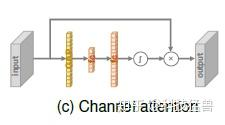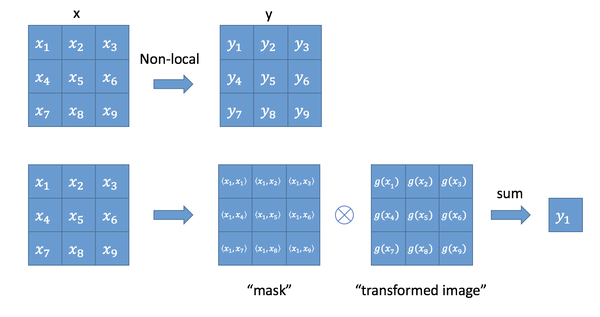• Gaussian: $f(\mathbb{x}_i, \mathbb{x}_j)=e^{\mathbb{x}_i^T \cdot \mathbb{x}j}, \mathcal{C}(x)=\sum{\forall j} f(\mathbb{x}_i, \mathbb{x}_j)$
• Embedded Gaussian: $f(\mathbb{x}_i, \mathbb{x}_j)=e^{\theta(\mathbb{x}_i)^T \cdot \phi(\mathbb{x}j)}, \mathcal{C}(x)=\sum{\forall j} f(\mathbb{x}_i, \mathbb{x}_j)$，其中 $\theta(xi)=W{\theta}x_i$ 和 $\phi(xi)=W{\phi}x_i$ 为两个embedding。

1.6.4 Dense Connections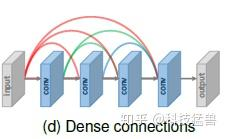1.6.5 Multi-path Learning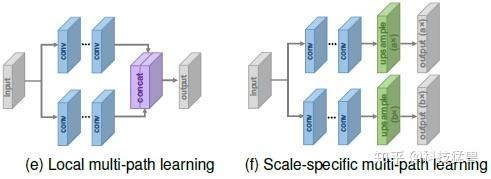Multi-path Learning是指通过模型的多个路径传递特性，这些路径执行不同的操作，以提供更好的建模能力。具体来说，它可以分为以下3种类型：

Global Multi-path Learning：

LapSRN

Local Multi-path Learning：

MSRN采用了一种新的多尺度特征提取块，如上图所示，在该块中，采用核大小为3×3和5×5的两个卷积运算同时提取特征，然后将输出拼接起来，再次进行相同的运算，最后再进行一个额外的1×1卷积。skip-connection通过elementwise加法连接输入和输出。通过这种局部多路径学习，SR模型可以更好地从多个尺度提取图像特征，进一步提高性能。

Scale-specific Multi-path Learning：

1.6.6 Advanced Convolution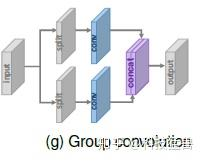Dilated Convolution. 空洞卷积： 增大感受野，有助于生成逼真的细节

Group Convolution：分组卷积： 可以在little performance loss的情况下减少大量的参数和运算，而CARN-M在性能损失很小的情况下将参数数量减少了5倍，运算减少了4倍。

1.6.8 Pyramid Pooling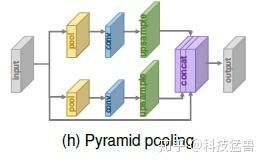Pyramid的思路就是把不同size的feature concat在一起。对于尺寸是 $h\times w\times c$ 的feature map，每个channel被划分成 $M\times M$ 块并通过GAP全局池化，生成 $M\times M\times c$ 的结果。再通过 $1\times1$ 卷积把输出压缩为single channel。之后，再经过双线性插值把结果进行上采样到原来feature map的维度。当 $M$ 变化时，模块结合全局和局部的上下文信息以提升性能。

### 1.7 常见的损失函数

• Pixel Loss：

$$L{pixel{l1}}(I,\hat I)=\frac{1}{hwc}\sum{i,j,k}|{\hat I{i,j,k}- I_{i,j,k}}|\tag{16}$$

$$L{pixel{l2}}(I,\hat I)=\frac{1}{hwc}\sum{i,j,k}({\hat I{i,j,k}- I_{i,j,k}})^2\tag{17}$$

• Content Loss：希望生成的图片从视觉上看更像是真实的图片

$$L_{content}(\hat I,I,\phi,l)=\frac{1}{h_lw_lcl}\sqrt{\sum{i,j,k}{(\phi{i,j,k}^{(l)}(\hat I))-(\phi{i,j,k}^{(l)}(I))^2}}\tag{18}$$

$\phi$ 是特征提取网络，往往是分类网络，用来提取网络的高维特征。

content loss代表2副图像高维特征之间的欧氏距离。它把分类网络引入超分中，不再关注pixel之间的匹配，而是使生成的图片从视觉上看更像是真实的图片。

• Texture Loss：希望重构的图片与原图有相同的风格

$$G_{ij}^{(l)}(I)=vec(\phi_i^{(l)}(I))\cdot vec(\phi_j^{(l)}(I))\tag{19}$$

$$L_{texture}(\hat I,I,\phi,l)=\frac{1}{cl^2}\sqrt{\sum{i,j,k}{(G{i,j}^{(l)}(\hat I))-(G{i,j}^{(l)}(I))^2}}\tag{20}$$

• Total Variation Loss：希望生成1的图片更平滑

$$L{TV}(\hat I)=\frac{1}{hwc}\sqrt{\sum{i,j,k}{(\hat I{i,j+1,k}-\hat I{i,j,k})^2 + (\hat I{i,j,k+1}-\hat I{i,j,k})^2}}\tag{21}$$

• Adversarial Loss：希望重构的图片与原图有相同的风格

$$L_{gan_ce_g}(\hat I; D)=-\log D(\hat I)\tag{22}$$

$$L_{gan_ce_d}(\hat I,I_s; D)=-\log D(I_s)-\log(1-D(\hat I))\tag{23}$$

$$L_{gan_ce_g}(\hat I; D)=(D(\hat I)-1)^2\tag{24}$$

$$L_{gan_ce_d}(\hat I,I_s; D)=(D(I_s)-1)^2+(D(\hat I))^2\tag{25}$$

ESRGAN进一步使用了：relativistic GAN作为GAN Loss：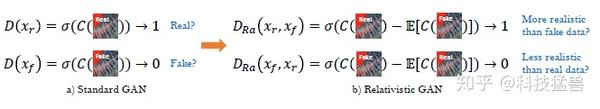C为判别器的输出， $\sigma$ 是sigmoid函数，ESRGAN取得不是判别器的输出，而是输出的相对值Ra。

$$LD^{Ra}=-E{xr}[\log(D{Ra}(x_r,xf))]-E{xf}[\log(1-D{Ra}(x_f,x_r))]\tag{26}$$

$$LG^{Ra}=-E{xr}[\log(1-D{Ra}(x_r,xf))]-E{xf}[\log(D{Ra}(x_f,x_r))]\tag{28}$$

## 2 渐进式方法1：Laplacian金字塔超分辨率网络

TPAMI 2018： Fast and accurate image super-resolution with deep laplacian pyramid networks (Ming-Hsuan Yang团队)

• 在多个金字塔级别上逐步重建高分辨率图像的子带残差。
• 利用recursive layer在金字塔级别之间和内部共享参数，从而大大减少了参数的数量。

• Parameter sharing
• Local skip connections
• Multi-scale training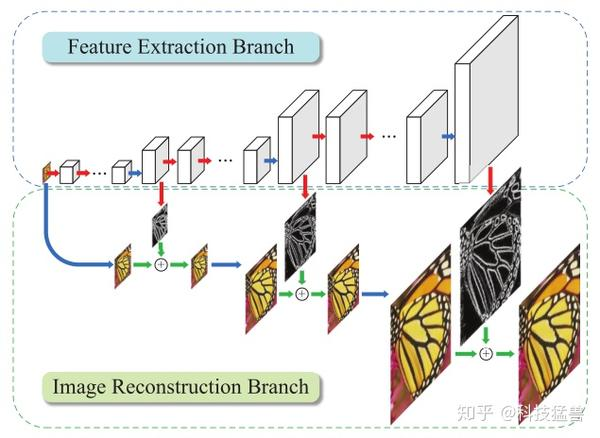• 特征抽取分支：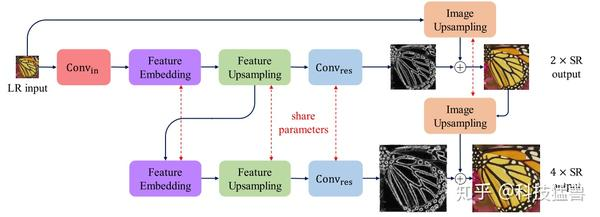Feature embedding的作用是上采样之前的特征提取。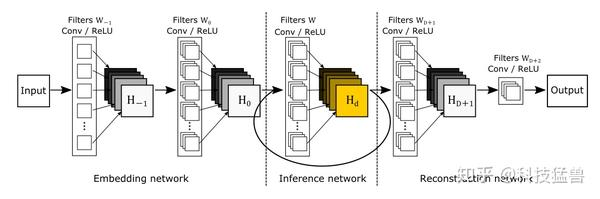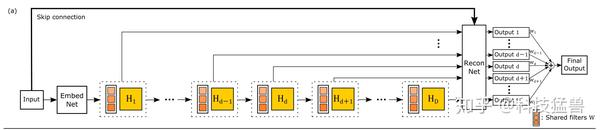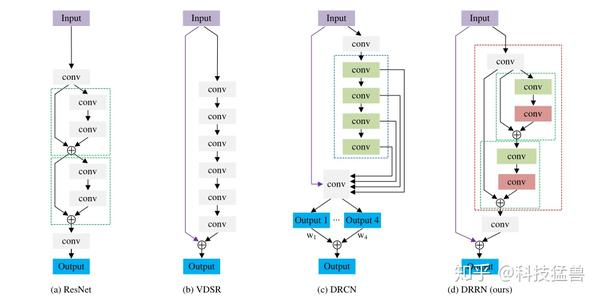DRCN和DRRN的结构分别如图7和图8所示：

DRRN(图8.d)直接共享Block的参数，一个Block是2个conv，128个filter。identity分支都来自first block的输出，如此多的残差连接很好地解决了梯度消失和梯度爆炸的问题，因为梯度在反向传播时存在多个路径。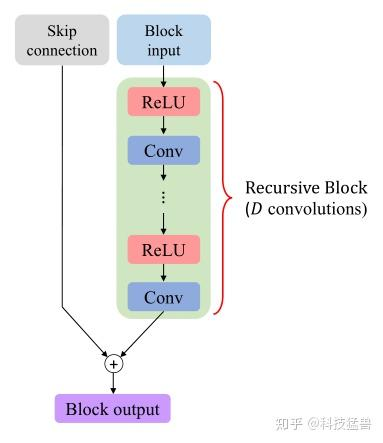$$depth=(D\times R+1)\times L+2\tag{1}$$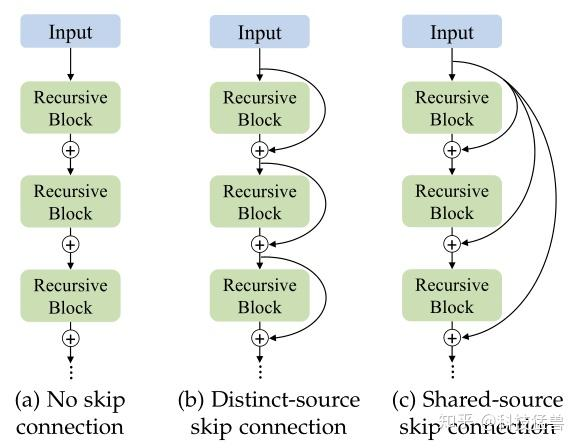• 图像重构分支：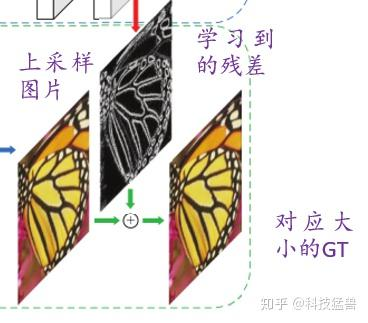• 损失函数：

Charbonnier Loss的表达式如下所示：

$$Charbonnier_Loss=\sqrt{x^2+\epsilon^2}\tag{2}$$

$$LS(y,\hat y;\theta)=\frac{1}{N}\sum{i=1}^{N}\sum_{l=1}^{L}{\rho(y_l^{(i)}-\hat yl^{(i)})}=\frac{1}{N}\sum{i=1}^{N}\sum_{l=1}^{L}{\rho(y_l^{(i)}-x_l^{(i)}-\hat r_l^{(i)})}\tag{3}$$

$$L(y,\hat y;\theta)=\sum_{S={2,4,8}}^{}L_S(y,\hat y;\theta)\tag{4}$$

• 对比实验：

1 模型设计对比：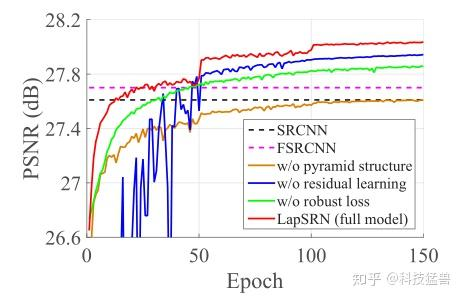Pyramid structure： 作者对比了去除金字塔结构但保留Global Residual learning。结果如棕色曲线所示，性能变差许多。

Global Residual learning： 作者对比了去除全局残差连接的模型。结果如蓝色曲线所示，发现收敛缓慢，并且在训练期间波动很大。

$L_2$ 损失函数： 作者对比了使用$L_2$ 损失函数的模型。结果如绿色曲线所示。

multi-scale training： 作者对比了不使用多重损失函数来监督每个金字塔级别的中间输出，结果如下图13所示：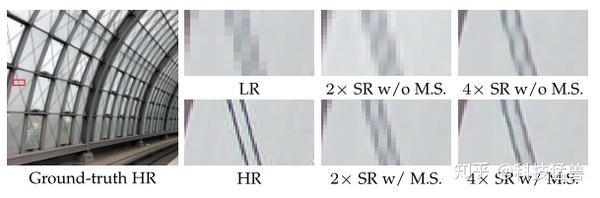2 参数共享对比：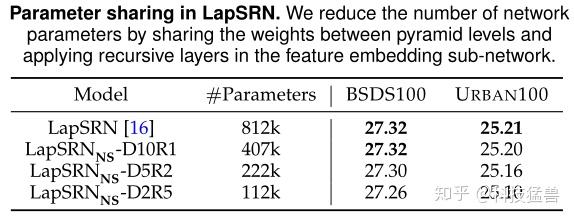LapSRN-D10R1(Recursive=1, conv=10)代表不在不同尺度之间的共享参数，也不在同一尺度下共享参数。

LapSRN-D5R2(Recursive=2, conv=5)代表不在不同尺度之间的共享参数，但在同一尺度下的2个Recursive Block之间共享参数。

LapSRN-D2R5(Recursive=5, conv=2)代表不在不同尺度之间的共享参数，但在同一尺度下的5个Recursive Block之间共享参数。

3 更深的模型对比：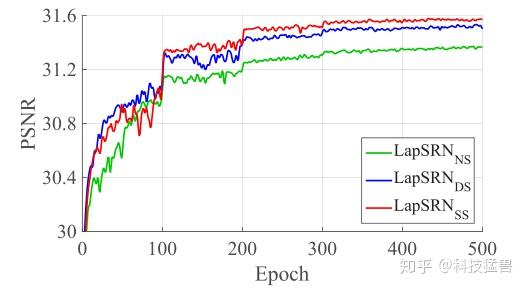$D$ 和 $R$ 数量的影响： 前文提到 $R$ 代表的是Recursive Block的数量，$D$代表的是每个Recursive Block中的卷积层数量。不同的Recursive Block之间的参数是共享的。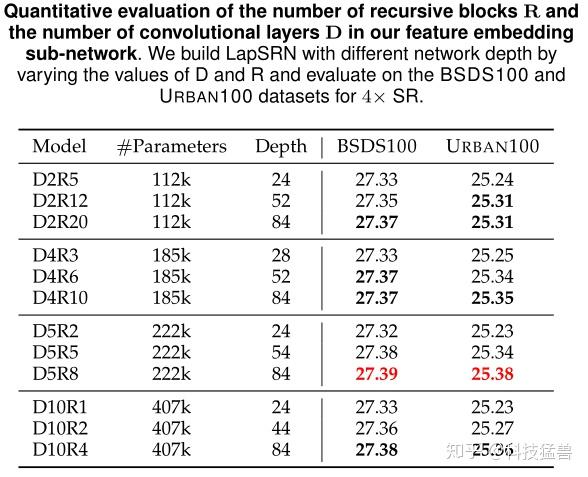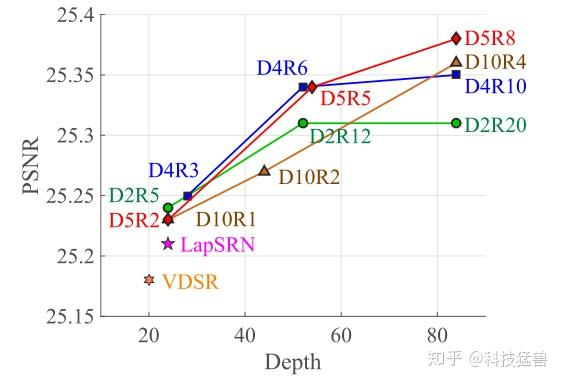## 3 渐进式方法2：ProSR：完全渐进式超分辨率网络

CVPR 2018： A Fully Progressive Approach to Single-Image Super-Resolution

• 在2018年的SISR挑战赛中，ProSR在SSIM方面排名第2，在PSNR方面排名第4。
• 运行速度比Top-ranking模型快5倍。

• 区别1：Asymmetric Pyramid Structure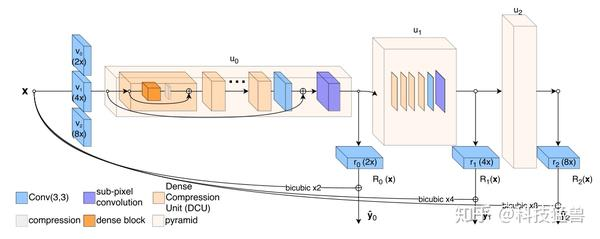• 区别2：标签

• 区别3：Dense Compression Units

$$BN-ReLU-Conv1\times1-BN-ReLU-Conv3\times3$$

$$Conv1\times1-ReLU-Conv3\times3$$

• 区别4：GAN的训练方法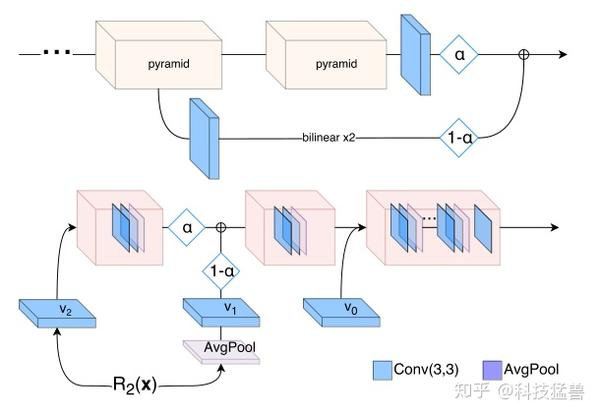$$\hat y=R_2(x)+\varphi_2(x)\tag{5}$$

$$L_{D_s}^{i}=(D_s(\hat r_i^s))^2+(D_s(r_i^s)-1)^2\tag{6}$$

Generator的损失函数可以写成：

$$L_{R_s}^{i}=(D_s(\hat ri^s)-1)^2+\sum{k\in\left{ 2,4 \right}}^{}{||\Phi_k(\hat y_i)-\Phi_k(y_i)||^2}\tag{7}$$

• 区别5：Curriculum Learning

Curriculum Learning是通过逐渐增加学习任务的难度来提高培训的策略。它通常用于序列预测任务和序列决策问题，在这些问题中可以获得训练时间的大幅加速和泛化性能的提高。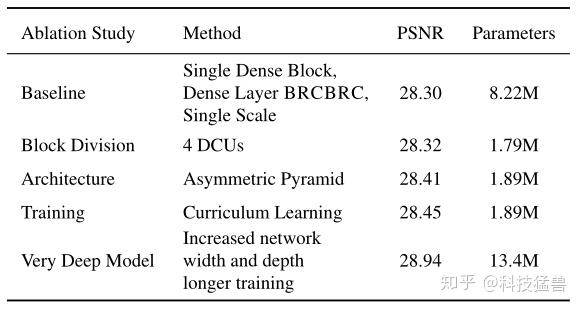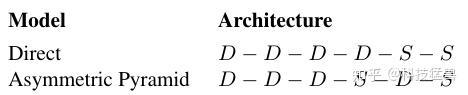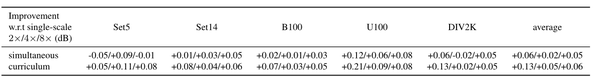$$\hat y^s=\hat r^s+\varphi_2(\hat y^{s-1})\tag{9}$$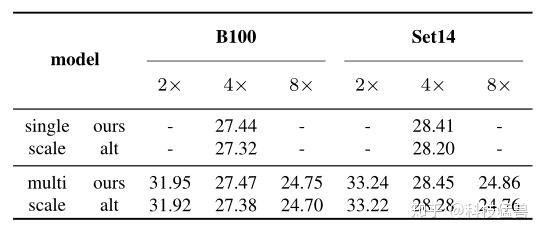## 总结

Progressive upsampling Super-resolution的基本思想主要是2点：

• Feature embedding与UpSampling交替进行。
• 主干网络输出的是残差，与插值结果进行相加操作得到最终的输出结果。

 TPAMI 2018：Fast and accurate image super-resolution with deep laplacian pyramid networks (Ming-Hsuan Yang团队)

 CVPR 2018：A Fully Progressive Approach to Single-Image Super-Resolution

 TPAMI 2020：Deep Learning for Image Super-resolution: A Survey